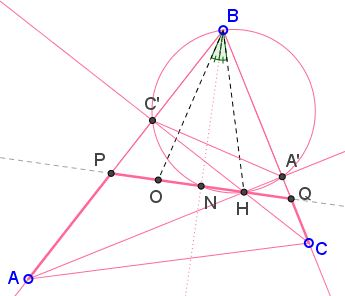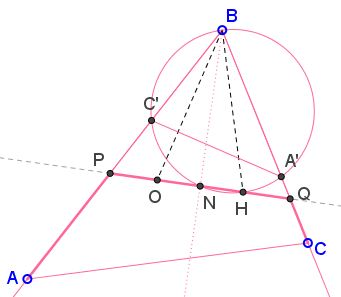# Euler Line Cuts Off Equilateral Triangle

### Problem

The applet may suggest that

In a triangle with an angle of $60^{\circ},\;$ the Euler line cuts off an equilateral triangle.The problem comes from a Russian online journal, mat.1september.ru/2000/no43_1.htm.

For one solution, among other related facts, see a separate page.

### Reformulation### Solution

$\mathbf{If \;\angle B=60^{\circ}\; then\; HO\perp \ell_B}$

Let $O\;$ be the circumcenter, $H\;$ the orthocenter, of $\Delta ABC.\;$ Let $\ell_B\;$ be the internal angle bisector at $B,\;$ and let it cross $OH\;$ at $N.$First, recollect that $H\;$ and $O\;$ are isogonal conjugate, meaning, in part, that $\angle OBN=\angle HBN.\;$ To establish the claim suffice it to show that $\Delta OBH\;$ is isosceles.

Let $AA'\;$ and $CC'\;$ be the two altitudes of $\Delta ABC.\;$ $A'C'\;$ is an antiparallel to $AC,\;$ such that the triangles $ABC\;$ and $A'BC'\;$ are similar. Now, in $\Delta AA'B,\;$ angle at $A\;$ is $30^{\circ}\;$ so that $AB=2A'B.\;$ It follows that the two triangles are similar with a coefficient of $2.\;$ In particular, the circumradius of $(ABC)\;$ is twice as large as that of $(A'BC').\;$ But, since $AA'\perp BC\;$ and $CC'\perp AB,\;$ $BH\;$ is a diameter of $(A'BC')\;$ and equals the radius $BO\;$ of $(ABC).$

$\mathbf{If\;HO\perp \ell_B\; then\; \angle B=60^{\circ}}$

We just reverse the argument.Since $O\;$ and $H\;$ are isogonal conjugate and $OH\perp\ell_B,\;$ $\Delta OBH\;$ is isosceles: $BH=BO.\;$ But $BO\;$ is the radius of $(ABC),\;$ whereas $BH\;$ is a diameter of $(A'BC').\;$ Thus, the coefficient of similarity of the two triangles is $2,\;$ and, in particular, $2A'B=AB,\;$ such that $\angle ABA'=60^{\circ}.$

### Acknowldegment

The reformulation above and the proofs are due to Ignacio Larrosa Cañestro and is a shortened translation of his web page.